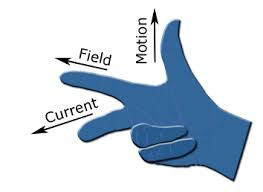Difference Between Fleming's Right hand and Left hand Rule - Electrical Diary -->

# Difference Between Fleming's Right hand and Left hand Rule

When a current-carrying conductor is kept in a magnetic field then this current-carrying conductor experiences a force perpendicular to the plane in which these two parameters magnetic field and current-carrying conductor exist. To determine the direction of the force on the current-carrying conductor fleming's left-hand rules are used. this principle is used in an electric motor.

When there is a relative motion between magnetic field and conductor then an emf is induced across the terminal of the conductor, according to faraday's law of electromagnetic induction. In this case, fleming's right-hand rule is used to determine the direction of the flow of current. This principle is used in the generator.

### Now Discuss Fleming's Left hand and Right-hand Ruleimage source Wikipedia

if we stretched our right hand's three-finger i.e (thumb, forefinger, and middle finger) in such a way that this three-finger are separated at perpendicular to each other. then if the thumb is pointed toward the motion of the conductor, the forefinger pointed toward the magnetic field then the middle finger will give the direction of the current flow.image source Wikipedia

if we stretched our left hand's three-finger i.e (thumb, forefinger, and middle finger) in such a way that this three-finger are separated at perpendicular to each other. then if the middle finger is pointed toward the direction of the current, the forefinger pointed toward the magnetic field then the thumb will give the direction of the force experienced by the current-carrying conductor.